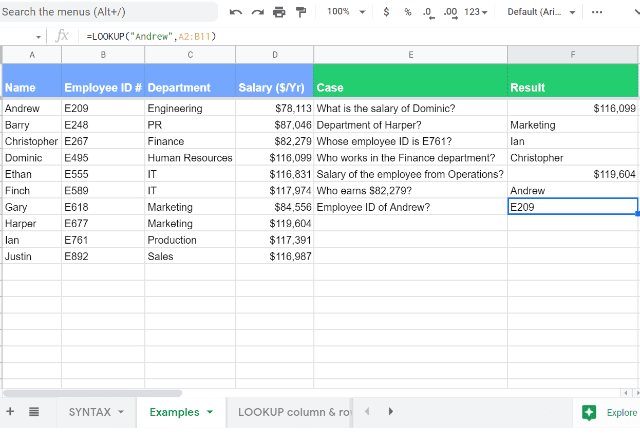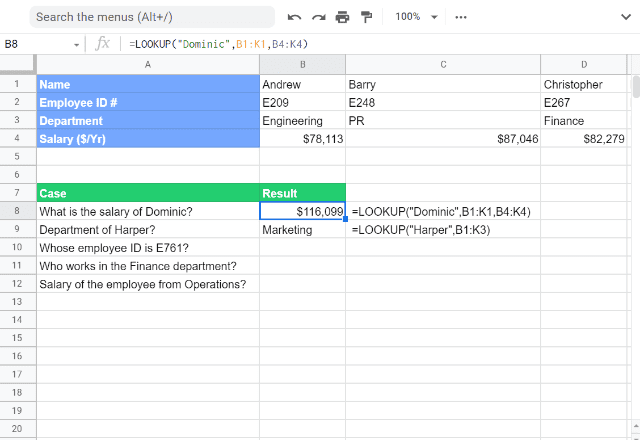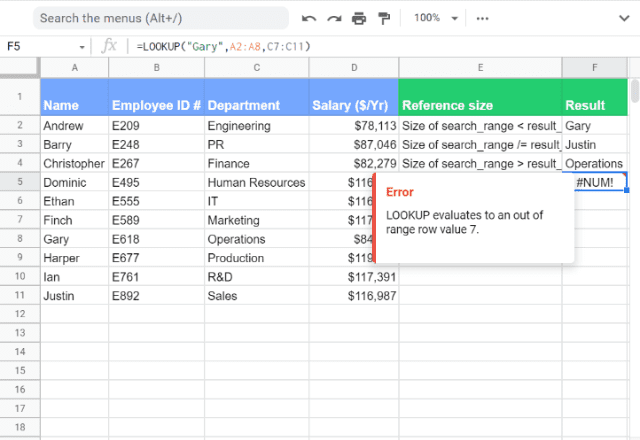# How to use the Google Sheets LOOKUP functionThe Google Sheets LOOKUP function searches through a row or column for a key and returns the value of the cell in a result range located in the corresponding position to the search row or column. Like VLOOKUP and HLOOKUP, LOOKUP allows you to retrieve specific data from your spreadsheet. However, this formula has two distinct differences:

• LOOKUP formula only works if the data in the column or row is sorted.
• While VLOOKUP only searches vertically (in a column) and HLOOKUP only searches horizontally (in a row), LOOKUP formula can search both.

You can use LOOKUP in two different ways.

### Option 1:

#### Syntax

LOOKUP(search_key, search_range, result_range)

This option allows you to search for a key in a search range (e.g. A3: A12) to return a result from a result range in the same position (e.g. F3: F12).

• search_key is the value that LOOKUP formula uses to search for the lookup.
• search_range is the range of cells in which the function will search for the search_key.
• result_range is the range of cells from which the function will pull the result.

### Option 2:

Syntax
LOOKUP(search_key, search_result_array)

=LOOKUP(search_key, search_result_array)

This option allows you to search for a key in the first row or column to return a result from the last row or column in the array.

search_result_array is the range you want to return a result from (e.g. A3: G15).  It will search the first row or column in the array and pull the result from the last row or column. If the array contains more (or an equal number of) columns than rows, the search will work vertically, searching the first column and pulling a result from the last column. If the array contains more rows than columns, the lookup will run horizontally within the rows.

### How to use Google Sheets LOOKUP Formula

I’ve applied the LOOKUP function to the data below and provided a few different examples of how to use it.### How to apply LOOKUP to a column

It’s worth noting that LOOKUP formula, unlike VLOOKUP and HLOOKUP formulas, can return values before the lookup column. Take a look at rows 4 and 5 to see examples of this.

You will also see that for the search_result_array option, the results come from the last row or column in the range. You can see this in the examples in rows 3 and 6. If you need to fetch results from a column or row in the middle of the range, you may have to use the VLOOKUP or HLOOKUP formulas.

One underlying assumption in all of the examples, from rows 2 through to 6, is that the columns are sorted in ascending order.

What if they are not? How does LOOKUP formula behave in this instance?

I’ve experimented with that in row 7. You’ll notice that the Salary column is not sorted. While the formula itself has returned a value, it’s incorrect. For the LOOKUP formula to function as expected, you need the lookup column to be sorted in ascending order.

Sometimes you might find that the lookup value does not match any of the values in the lookup column or row (the last example in the screenshot above). In this scenario, the formula hooks onto the nearest and smallest value for a match. This may not always make practical sense, and you might need an exact match. This is where this formula falls short, and you need to rely upon VLOOKUP and HLOOKUP for exact matching capabilities. Unlike those functions, LOOKUP function does not have an option for approximate vs. exact matches.

OK, so that was an example for the columns. The image below shows you some examples of how to use LOOKUP function on rows.

### How to apply LOOKUP to a rowHere the same logic applies, but you select ranges as rows instead of columns. You can use either syntax option, and just like in the previous example, you must sort your data in ascending order beforehand.

#### LOOKUP Formula: Can it misbehave?

So far I have been experimenting with equal-height columns or equal-width rows. What happens if I input a different sized reference to the search_range and the result_range?You can see from the examples that different sized ranges do not cause an issue as long as the relative position of the matched value from the search_range does not exceed the size of the result_range.

I only get an error in the last example, where the result range is not large enough to match the row number of the search key in the search_range.

In the second and third examples, you can see that I don’t get an error, but the answers are wrong. This is because the search_range and result_range do not align.

In the second example, the function determines that the item it’s searching for is in the fifth row of the search_range. It then pulls the result from the fifth row of the result_range.

Because the ranges are not aligned, the result that the function fetches is wrong. This can be seen in the third example as well.

### Comparison of LOOKUP, VLOOKUP, and HLOOKUP

I’ve explained some of the differences between the LOOKUP function and its counterparts, the VLOOKUP and HLOOKUP functions. Now that you’ve seen some examples and have a better idea of the way LOOKUP is used, I will summarize the differences, advantages, and disadvantages of each.

The LOOKUP function is more flexible than VLOOKUP or HLOOKUP. There are two main reasons for this.

Firstly, the LOOKUP function works in either a vertical or horizontal arrangement. In contrast, you can only use the other functions for vertical (VLOOKUP) or horizontal (HLOOKUP) data layouts.

Secondly, LOOKUP function is more flexible due to its ability to search columns or rows that are anywhere on the spreadsheet. The VLOOKUP and HLOOKUP functions require the search column to be the left-most column in the data set, which can be very inconvenient.

The main drawback of the LOOKUP function is that it only works if the data is sorted. Furthermore, because the LOOKUP function can give erroneous results if the data is unsorted (instead of giving an error message which would alert you to the problem), there is a risk that you could get incorrect results from the LOOKUP function without realizing.

This can also be the case with LOOKUP’s other drawback: its tendency to give approximate matches when no exact match is found.

#### Key tips when using LOOKUP in Google Sheets:

When you are using the search_result_array option, if your range contains more columns than rows, LOOKUP will search from left to right over the first row of the range. This works like a horizontal lookup.

If your search_key is not found, the lookup will use the closest matching key in the range with a lower value. For example, if your search_key is 7 but your dataset contains 4,5,6,8 and 9, the lookup will use 6 as the search_key.

That’s how to get started with the LOOKUP function in Google Sheets!

If you need help sorting your data, check out our post on how to use the SORT function.

#### Did you like this post?

Share these spreadsheet tips with your colleagues and friends via the social media buttons on the left!

Editor’s note: This is a revised version of a previous post that has been updated for accuracy and comprehensiveness.